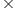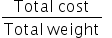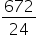Maths-
General
Easy

Question

# The cost of 16 bags of washing powder, each weighing 1.5 kg, is Rs.672. Find the cost of 18 bags of the same, each weighing 2 kg.Hint:

## The correct answer is: Rs.1008.

### Step 1 of 2:It is given that cost of 16 bags of washing powder, each weighing 1.5 kg, is Rs.672.Total weight of washing powder that will cost Rs.672 = No. of bagsWeight of each bag= 161.5 = 24 kgCost of 1 kg of washing powder === 28Step 2 of 2:Now we are asked to find the cost of 18 bags of the same, each weighing 2 kg.Total weight of washing washing powder = 218 = 36 kgTotal cost = Total weightCost of 1 kg= 3628 = Rs.1008Final Answer: Hence, the cost of 18 bags of the same, each weighing 2 kg is Rs.1008.#### With Turito Foundation.#### Get an Expert Advice From Turito.